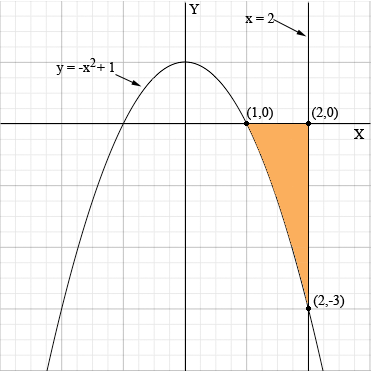SEARCH HOMEMath Central Quandaries & QueriesQuestion from Rogerson, a student: Please help me with this. Find the area, S, of the shaded region enclosed by the given curve, the given line and the x-axis. y = -x^2 + 1 line x = 2 thanks.Rogerson,

I sketched the curve y = -x2 + 1 and the line x = 2 and shaded the region in the plane bounded by these two curves and the x-axis.The shade region is entirely below the x-axis and hence if you integrate -x2 + 1 for x between 1 and 2 the value will be negative. The area of the shaded region is then minus this value.

HarleyMath Central is supported by the University of Regina and The Pacific Institute for the Mathematical Sciences.